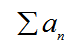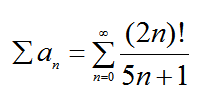# What are the Ratio and Root Tests? Do they always give you the information you need to determine convergence or divergence? Give examples.

Question
3 views

What are the Ratio and Root Tests? Do they always give you the information you need to determine convergence or divergence? Give examples.

check_circle

Step 1

A mathematical series can be convergent or divergent subject to certain conditions. Ratio test and the Root test are two of the many tests used to establish the convergent or divergent behaviour of the series.

Step 2

The definition of ratio test is as follows:

Suppose we have a series of the form:Let us define a limit L such that

Step 3

For example suppose we have the following series to test for convergence:And we want to apply the ratio test to this series, for this we will calculate the limit L as shown below:

...

### Want to see the full answer?

See Solution

#### Want to see this answer and more?

Solutions are written by subject experts who are available 24/7. Questions are typically answered within 1 hour.*

See Solution
*Response times may vary by subject and question.
Tagged in

### Other